Refer to our Texas Go Math Grade 3 Answer Key Pdf to score good marks in the exams. Test yourself by practicing the problems from Texas Go Math Grade 3 Unit 1 Assessment Answer Key.

Vocabulary

Choose the best term from the box to complete the sentence.

Question 1.
The ___________ states that you can add two or more nLHllhers in any order and get the same sum. (p. 113)
Explanation:
The commutative property of whole numbers states that “The sum and the product of two whole numbers remain the same even after interchanging the order of the numbers”.

Question 2.
The _____ tells how many parts are being counted. (p. 49)
Explanation:
The bottom number (denominator) tells how many parts the whole (one) was divided into.
The top number (numerator) tells how many of the parts to count. says, “Count one of two equal parts.” says, “Count three of four equal parts.”

Concepts and Skills

Go Math Expressions Grade 3 Unit 1 Assessment Answer Key Question 3.
Write the number in standard form. TEKS 3.2.A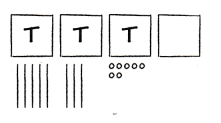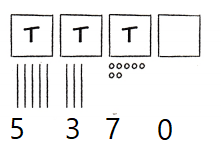Question 4.
Draw a quick picture to show 12,527. TEKS 3.2.A.

Write the value of the underlined digit. TEKS 3.2.B

Question 5.
9,654
Explanation:
6 is on 100th place. So value of 6 is 600

Question 6.
78,120
Explanation:
7 is on 10,000 place. So value of 7 is 70,000

Question 7.
43,598
Explanation:
3 is on 1000 place. So value of 3 is 3000

Texas Go Math Grade 3 Unit 1 Answer Key Pdf Question 8.
27,846
Explanation:
4 is on 10th place. So value of 4 is 40

Write the fraction represented by the sum of unit fractions. TEKS 3.3.D

Question 9.
$$\frac{1}{6}$$ + $$\frac{1}{6}$$ + $$\frac{1}{6}$$ + $$\frac{1}{6}$$ = _____
Answer: $$\frac{4}{6}$$
Explanation:
$$\frac{1}{6}$$ + $$\frac{1}{6}$$ + $$\frac{1}{6}$$ + $$\frac{1}{6}$$ =  $$\frac{4}{6}$$

Question 10.
$$\frac{1}{3}$$ + $$\frac{1}{3}$$ = ___
Answer: $$\frac{2}{3}$$
Explanation:
$$\frac{1}{3}$$ + $$\frac{1}{3}$$ =  $$\frac{2}{3}$$

Estimate. Then find the sum or difference. TEKS 3.4.A, 3.4.B

Question 11.
Estimate: ___Explanation:
Sum of given two number is 769

Question 12.
Estimate: ___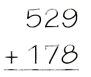Explanation:
Explanation:
Sum of given two number is 707

Texas Go Math 3rd Grade Unit 1 Assessment Answer Key Question 13.
Estimate: ___Explanation:
difference of given two number is 385
Question 14.
Estimate: ___Explanation:
difference of given two number is 128

Texas Test Prep

Fill in the bubble for the correct answer choice.

Question 15.
The Arkansas River is 1,443 miles long. The Rio Grande River is 1,759 miles long, and the Colorado River is 1,450 miles long. Which lists the river lengths from longest to shortest? TEKS 3.2.D
(A) 1,443; 1,759; 1,450
(B) 1,443; 1,450; 1,759
(C) 1,759, 1,450; 1,443
(D) 1,759; 1,443; 1,450
Explanation:
listed the river lengths from longest to shortest based on given length

Question 16.
Joshua walks $$\frac{7}{8}$$ of a mile to the park. Which point represents the distance Joshua walked? TEKS 3.3.BExplanation:
Z represents the $$\frac{7}{8}$$

Question 17.
Samantha earned the bills and coins shown below by taking her nephew to the zoo. How much money did Samantha earn? TEKS 3.4.C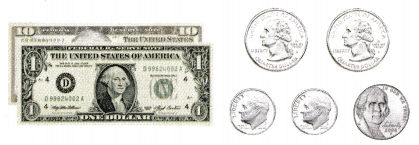(A) $11.75 (B)$1.75
(C) \$11.75
(D) Not here

Go Math Unit 1 Assessment Grade 3 Pdf Question 18.
Alyssa ordered a hoagie for lunch. The missing part shows how much she ate. How much of the hoagie is left? TEKS 3.3.C(A) $$\frac{2}{3}$$
(B) $$\frac{1}{4}$$
(C) $$\frac{1}{2}$$
(D) $$\frac{1}{3}$$
Answer: (D) $$\frac{1}{3}$$
Explanation:
As picture, she ate $$\frac{2}{3}$$ part. So left is
1 – $$\frac{2}{3}$$ = $$\frac{1}{3}$$

Texas Test Prep

Fill in the bubble for the correct answer choice.

Question 19.
A hot air balloon is 578 feet above the ground. Between which two numbers on the number line is 578? TEKS 3.2.C
(A) between 70 and 80
(B) between 400 and 500
(C) between 700 and 800
(D) between 500 and 600
Answer: (D) between 500 and 600
Eplanation:
578 is between  500 and 600

Question 20.
Grace baked a pan of brownies. On some of the pieces she added walnuts. Which fraction of the pan of
brownies has walnuts? TEKS 3.3.A(A) $$\frac{2}{6}$$
(B) $$\frac{6}{6}$$
(C) $$\frac{3}{6}$$
(D) $$\frac{3}{3}$$
Answer: (A) $$\frac{2}{6}$$
Explanation:
$$\frac{2}{6}$$ = $$\frac{1}{3}$$
Grace baked a pan of brownies. On some of the pieces, she added walnuts. so, we have 6 pieces and added walnuts in 3 pieces. So $$\frac{1}{3}$$ added.

Texas Go Math Grade 3 Volume 1 Pdf Unit 1 Test Answers Question 21.
Which is the best estimate for the total number of visitors to the tree farm on Saturday and Sunday? TEKS 3.4.BExplanation:

Question 22.
Ethan runs on a track that is $$\frac{3}{8}$$ mile long. He completes two laps. Which fraction is equivalent to the distance Ethan ran? TEKS 3.3.A, 3.3.B, 3.3. 3.3.G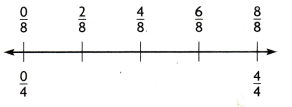(A) $$\frac{1}{4}$$
(B) $$\frac{3}{4}$$
(C) $$\frac{5}{8}$$
(D) $$\frac{4}{8}$$
Answer: (B) $$\frac{3}{4}$$
Explanation:
Ethan runs on a track that is $$\frac{3}{8}$$ mile long. He completes two laps. So
$$\frac{3}{8}$$ + $$\frac{3}{8}$$ = $$\frac{6}{8}$$ = $$\frac{3}{4}$$

Texas Test Prep

Fill in the bubble for the correct answer choice.

Question 23.
Mrs. Rosetti baked two pans of lasagna for a family dinner. There will be 5 adults and 3 children. If she plans to serve each person an equal share, how much lasagna will each person get? TEKS 3.3.E.(A) $$\frac{3}{8}$$
(B) $$\frac{5}{8}$$
(C) $$\frac{2}{8}$$
(D) $$\frac{8}{8}$$
Answer: (C) $$\frac{2}{8}$$
Explanation:
Total Family members (5 adults and 3 children) are 5 + 3 =8
Mrs. Rosetti baked two pans of lasagna and equally distributed as $$\frac{2}{8}$$

Go Math Unit 1 Assessment Guide Grade 3 Pdf Question 24.
Matthew ran $$\frac{5}{8}$$ mile during track practice. Paul ran $$\frac{5}{6}$$ mile. Which of the following correctly compares the fractions? TEKS 3.3.H
(A) $$\frac{5}{6}$$ = $$\frac{5}{8}$$
(B) $$\frac{5}{8}$$ < $$\frac{5}{6}$$
(C) $$\frac{5}{8}$$ > $$\frac{5}{6}$$
(D) $$\frac{5}{6}$$ < $$\frac{5}{8}$$
Answer: (B) $$\frac{5}{8}$$ < $$\frac{5}{6}$$
Explanation:

Question 25. Brady and Alexis are reading the same book. Brady has read $$\frac{2}{6}$$ of his book, and Alexis has read $$\frac{5}{6}$$ of her book. Which of the following correctly compares the fractions? TEKS 3.3.H
(A) $$\frac{5}{6}$$ > $$\frac{2}{6}$$
(B) $$\frac{2}{6}$$ = $$\frac{5}{6}$$
(C) $$\frac{2}{6}$$ > $$\frac{5}{6}$$
(D) $$\frac{5}{6}$$ < $$\frac{2}{6}$$
Answer: (A) $$\frac{5}{6}$$ > $$\frac{2}{6}$$

Texas Go Math Grade 3 Unit 1 Assessment Answer Key Question 26.
Taylor baked a cake to serve after dinner. Suppose she cut the cake into 8 equal-size pieces and 6 people ate all the pieces. Explain how Taylor could have divided the pieces so that everyone ate the same amount of cake. You may show your work in a drawing. TEKS 3.3E